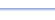Program: REGPOLY

REGPOLY

• Computes the area of a regular polygon, given the number of sides and either the radius, apothem, side length, or perimeter.

Useful for:

• SAT
• ACT
• Geometry
• Precalculus
• Trigonometry
• Calculus

See full list of programs

Example

Q: Find the area of the regular octagon:

Solution:

Run REGPOLY.  How?

Enter N = 8.  This is
the number of sides.

Choose 2 for 'Given
info'.  We are given
the apothem - the
distance from the
center to a side, at
a right angle.

Enter A = 4 for the
length of the apothem.

The program reports:

Interpretation: